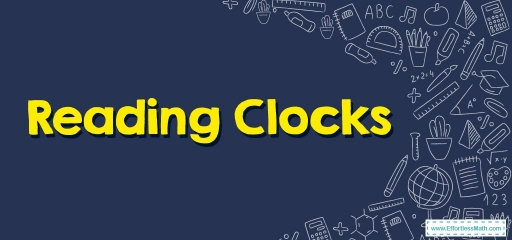Need complete information about the clock and how to read them? No need for searching any further! What you need is provided for you in this article.Clocks or timepieces are gadgets utilized for measuring and indicating the time.

Analog clocks are a device to show the time that has numerals all around the edge as well as hands that go around to show what time it is. Clocks are utilized to tell how much time has passed.

Reading clocks is a talent that is simple to learn by taking a bit of time and energy.

Analog clocks get divided up around a circle, if you read the minute and hour hand separately, it assists in seeing what time it is. With a bit of effort, you can learn how to read clocks easily.

## Way to Read a Clock

1- Learn the way clocks are divided. Clocks are divided into $$12$$ portions. On a clock’s top, you’ll find the “$$12$$.” On the right of this “$$12$$,” you’ll find a “$$1$$.” If you then follow the numerals from the right or go “clockwise,” they’ll read from “$$1$$” to “$$12$$.”

• The numerals which mark each area are the hours
• The areas in-between numerals are separated into five-minute portions. At times, there are also small lines running alongside the clock separating these portions.

2- Utilize the small hand for reading hours. Clocks come with two hands: a small hand and a large hand. The small hand marks hours. Whatever numeral it’s pointing to is the hour of the day.

• For instance, if the small hand points to a “$$1$$,” it is in the one o’clock hour.

3- Utilize the large hand for reading minutes. Use the numeral it’s pointing to, and then multiply it by five to find out the minutes. Whenever it’s pointing to a “$$12$$,” it’s at the top of the hour. Should the large hand have a mark in-between the numerals, count those marks, and after that add the marks to the minutes (clock number times five). For instance:

• Should the large hand be pointing to a “$$3$$,” it’s fifteen minutes after the hour.
• Should the large hand be pointing to a “$$12$$,” it’s at the top of the hour. Read whichever numeral the small hand is pointing to.
• Should the large hand be in-between “$$1$$” and “$$2$$,” see which dash it’s pointing to. For instance, if it’s on the third dash after the “$$1$$,” it’s $$8 ‘$$minutes after the hour. ($$1 × 5 +$$ the number of dashes).

4- Put all these together to find out the time. When you have figured out the hour and the minutes, then you will know the time. For instance:

• If the small hand points to “$$1$$” and the large hand points to “$$12$$,” it’s “one o’clock.”
• If the small hand points to “$$1$$” and the large hand points to “$$2$$,” it’s “one-ten” or “ten minutes after one.”
• If the small hand points to “$$1$$,” and the large hand is halfway in between the “$$2$$” and “$$3$$,” it’s about “one-twelve” or “twelve minutes after one.”

Note: Distinguish between AM and PM. AM and PM can’t be figured out on a regular clock. You must know what time of the day it is. From midnight to noon the following day, it is considered as AM. From noon to midnight, it is considered as PM.

• For instance, if it is early in the morning and the small hand points to “$$9$$” and the large hand points to “$$12$$,” it is “$$9$$ AM.”

### Reading Clocks – Example 1:

Write the time below the clock.

Solution:

As mentioned, the clock has two hands, a small hand, and a large hand. The small hand shows the clock and the large hand shows the minute. Here the small hand is on the number $$12$$ so the hour is $$12$$ and the large hand is on the number $$15$$ so $$15$$ minutes past from $$12$$. This indicates that it is $$12:15$$.

### Reading Clocks – Example 2:

Write the time below the clock.

Solution:

Here, the small hand is between two numbers: the number $$7$$ and the number $$8$$. So, the hour is $$7$$ and the large hand is on the number $$10$$ so $$10$$ minutes left until $$8$$ o’clock. This indicates that it is $$7:50$$.

Write the time below each clock.

1)

2)

1. $$\color{blue}{6:16}$$
2. $$\color{blue}{5:25}$$

### What people say about "Reading Clocks - Effortless Math: We Help Students Learn to LOVE Mathematics"?

No one replied yet.

X
30% OFF

Limited time only!

Save Over 30%

SAVE $5 It was$16.99 now it is \$11.99